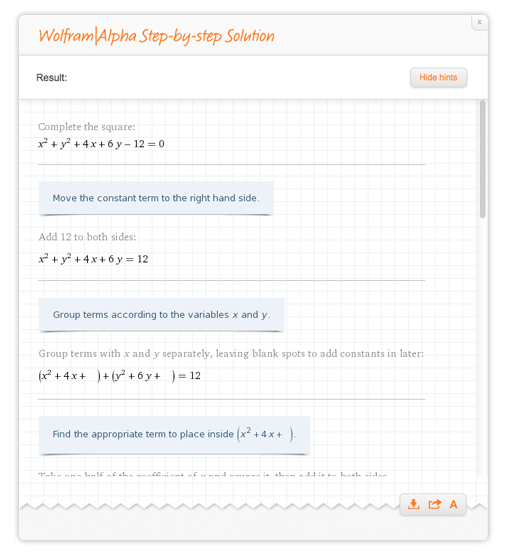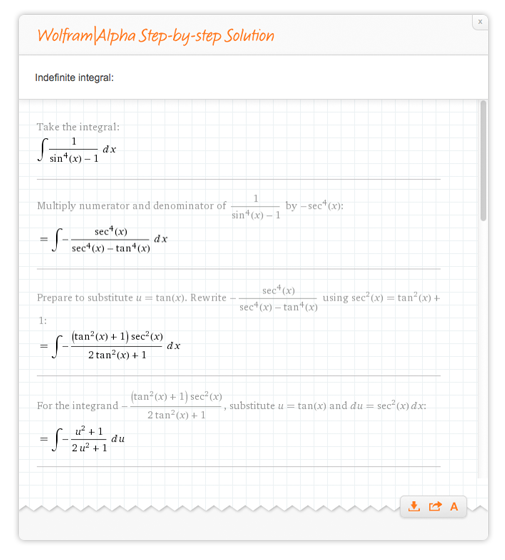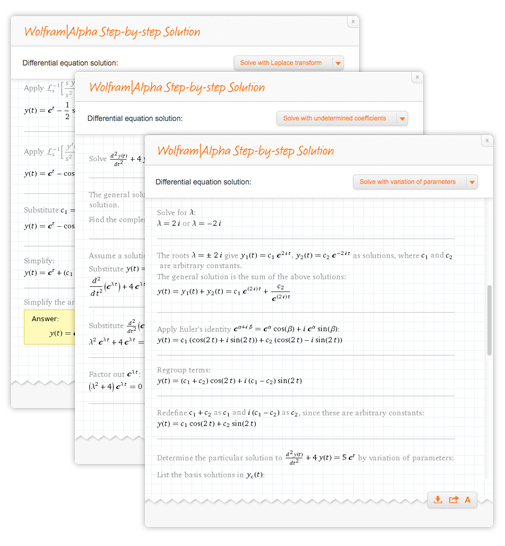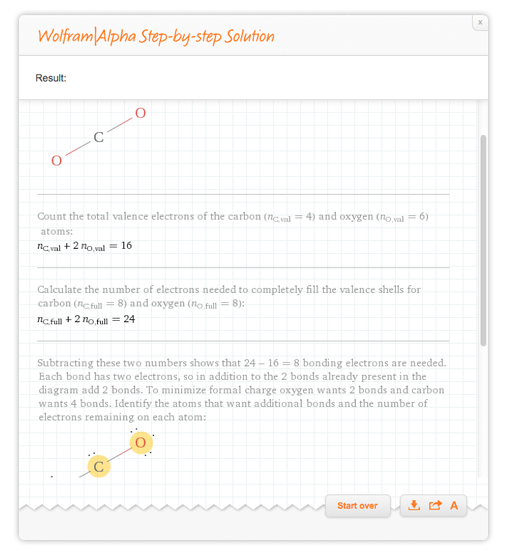# What Can Step-by-step Solutions Do?

January 15, 2014 —Comments Off

Wolfram|Alpha Pro has become well known for its ability to show not only the answer to a question, but also the Step-by-step solution to show how to find the answer. Since its first release, we have developed new features and content for Step-by-step solutions. We’ve added hints and the ability to walk through problems one step at a time, and we’ve added support to show multiple methods for solving problems whenever possible.

So, let’s have a look at what Step-by-step solutions can do now.

Need help with arithmetic? We offer multiple “methods” for illustrating basic arithmetic—visualize arithmetic using the number line, math manipulatives, and pie charts, or try writing it out with long arithmetic. In more complicated expressions, follow along through the proper order of operations. Here are some examples: 14 + 7, 20034 – 65, and 9 (3 + 1) + 17 / (6 – 12).If you’re learning about polynomials and rational functions, Wolfram|Alpha can help there, too. We could expand (x + 1) (x – 1) (x + 2), factor x^3 + 2x^2 + x + 2, complete the square x^2 + y^2 + 4x + 6y – 12 = 0, or simplify 1 / (1 + 1 / (1 + 1 / (1 + 1 / x))).Or perhaps you want to solve equations. How about we solve x^2 + 5x + 6 = 0:We can also work through calculus problems one step at a time. For instance, try finding the derivative of x / (x + 1), the limit of x*log(x) as x -> 0, or integrate 1/ (sin (x) ^4 – 1).If you want to solve ordinary differential equations, you can now do that with multiple methods.We believe so strongly in our Step-by-step solutions that we are developing them for areas outside of math. For example, we’ve started doing chemistry problems step-by-step:We’ve only scratched the surface of what Wolfram|Alpha can show steps for in this post. To see a full list of what we have to offer, check out our examples page. To suggest new features and content areas, please contact us, and stay tuned for new Step-by-step features as we continue to grow.

I love it, recommend it and think this will be the new studying way of 2014.

Posted by Jan van Willigen January 15, 2014 at 1:24 pm

When step by step solution will be implemented in series,for exemple?

Posted by Edoardo February 11, 2014 at 5:22 pm

Is there a way to force Wolfram-Alpha to display a step by step solution? Sometime I enter my formula and the step-by-step button is nowhere to be found.

Posted by Andrew Molder April 27, 2014 at 3:10 pm

Thank you for your question! We are continually working to add new step-by-step results to Wolfram|Alpha. Please submit feedback if we don’t have a step-by-step that you wish to see.

Posted by The Wolfram|Alpha Team April 29, 2014 at 3:48 pm

(9x+6):3+2=13

Posted by leandro May 26, 2014 at 2:48 pm

Please add some step-by-step help for problems such as factoring & simplifying problems like:
(3cos^2x+4cosx+1) / (cos^2-1)
I factored out the top and bottom, canceling like factors, and ended with
(3cosx+1) / (cosx-1)
I went to double check my answer to see if it could be simplified further and was told the final form is
(4/cosx-1)+3 … but no option for step-by-step was given to show how to go from (3cosx+1)/(cosx-1) to (4/cosx-1)+3. As a paying member I find it frustrating that I joined WolframAlpha to help me, yet it can’t even show me (what is more than likely a very obvious and simple, but I just can’t see it) the way they arrive at their final solution.

Posted by Mandy July 6, 2014 at 7:48 pm

Please,put the button step by step solution,because in this way al the students that don’t know how a mats ex. is resolving and don’t look only the results. And then we are all a pro customers,si we pay for this

Posted by gerardo October 29, 2014 at 5:53 pm

find a cubic function in the form of f(X)= ax^3+bx^2+cx+d that has a relative minimum at (0,0) and a relative maximum at (2,2)

Posted by Dawn March 25, 2015 at 4:44 pm

I can not find complete step by step solutions for solving simultaneous equations for 2 variables. There are steps missing that would require someone more knowledgable than the student to explain the missing steps. As a parent I feel ripped off because my child can not use this part independently.

Posted by Diane Beitz May 1, 2015 at 12:56 pm

Hi Diane, thank you for your comment. Could you please provide more feedback on what changes you would like to see?

Posted by The Wolfram Team May 15, 2015 at 9:34 am